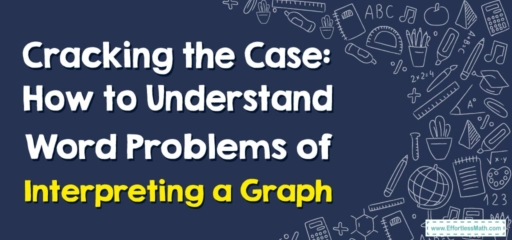# Cracking the Case: How to Understand Word Problems of Interpreting a Graph

Hello, math detectives!

Our mission today, should you choose to accept it, is to solve the enigma of word problems of interpreting a graph. Like keen detectives, we'll be deciphering clues, drawing conclusions, and, most importantly, cracking the case wide open!## 1. The Evidence: Graphs

Our main evidence in these cases is a graph. It may be a line graph, a bar graph, a pie chart, or a scatter plot. The graph presents us with visual data that we need to understand and interpret.

## 2. The Case: Word Problems

The word problems present us with a scenario. Our task? Use the information in the graph to answer the questions posed in the problem.

### Your Detective Toolkit: Interpreting Graph Word Problems

Here’s how we crack the case:

#### Step 1: Examine the Evidence

Firstly, look closely at the graph. What kind of graph is it? What data is being presented? What does each axis or segment represent?

#### Step 2: Understand the Case

Read the word problem. What is it asking? What information do you need from the graph to solve the problem?

#### Step 3: Crack the Case

Use the information from the graph to answer the question in the problem. Let’s consider this case: The line graph shows the temperature changes throughout the day, with time on the $$x$$-axis and temperature on the $$y$$-axis. If the graph shows the temperature at $$6\ PM$$ as $$20°C$$, what was the temperature change since noon if the temperature at noon was $$15°C$$?

1. Examine the Evidence: It’s a line graph showing temperature changes over time.
2. Understand the Case: We need to find out the temperature change from noon to $$6\ PM$$.
3. Crack the Case: If the temperature was $$15°C$$ at noon and $$20°C$$ at $$6\ PM$$, then it increased by $$20\ – 15 = 5°C$$.

And just like that, we’ve cracked another case! Keep honing your detective skills with more word problems of interpreting graphs, and you’ll become the Sherlock Holmes of the math world. Until our next case, keep your detective hat on and your magnifying glass at the ready!

### What people say about "Cracking the Case: How to Understand Word Problems of Interpreting a Graph - Effortless Math: We Help Students Learn to LOVE Mathematics"?

No one replied yet.

X
30% OFF

Limited time only!

Save Over 30%

SAVE $5 It was$16.99 now it is \$11.99